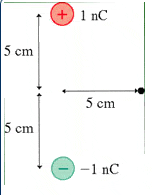# Strength of magnetic field

I've been working on this problem for awhile now, and I cannot seem to grasp it.

In this problem, we are to find the strength and direction of the electric field at the point indicated by the black dot (see image below). How would I go about doing this?Thanks in advance!!Last edited:

## Answers and Replies

jtbell
Mentor
Hint: Stationary electric charges don't produce magnetic fields.Whoops, I meant electric field. Silly me :P

jtbell
Mentor
I kind of suspected it was supposed to be electric field, but I just wanted to make sure. Textbook authors and professors do sneaky tricks sometimes.First, find the electric field at the black dot, that is produced by the positive charge alone. Ignore the negative charge for the moment.

Second, find the electric field at the black dot, that is produced by the negative charge alone. Ignore the positive charge for the moment.

Third, add together the two electric fields that you just found. This gives you the electric field at the black dot, that is produced by the two charges together.

Hint: electric field is a vector quantity, so the result of each step has both magnitude and direction.

I've tried that a million times and I'm still not coming out with the right answer >:-O By following that procedure, my answer keeps coming out to zero. I got ~1800 N/C for the field of the positive charge and ~-1800 N/C for the field of the negative charge... What am I doing wrong????

jtbell
Mentor
I'll repeat my hint: the electric field is a vector. A vector has magnitude and direction. You have the magnitude of the field produced by each charge alone, except that the magnitude of a vector is always positive, so get rid of the minus sign on the field produced by the negative charge.

What is the direction of the electric field produced by the positive charge alone, and what is the direction of the electric field produced by the negative charge alone?

Do you remember how to add vectors that are in different directions?

Okay, I think that makse sense... let's see if I'm on the right track.

So I figured the magnitude of each vector to be 1800 N/C. The direction of the field produced by the positive charge would then be -1800cos(45)i+1800sin(45)j and the direction of the field produced by the negative charge would be 1800cos(45)i+1800sin(45)j. (45 is in degrees)

To add the vectors, I would just add each of the i components and each of the j components to get 2545j (the i components cancel out)... correct? But this would mean that the vector is in the horizontal direction... and when I put 0 degrees in for the answer, it keeps telling me I'm wrong.

Grr... I wish the professor actually spent time on these things...

Thanks so much for your help/patience... it means a ton!

Gokul43201
Staff Emeritus
Science Advisor
Gold Member
What direction is $\hat{j}$ along ?

Last edited:
oh, i had them backwards. grr. I guess that would mean that j would be along the x-axis, making that whole thing a bunch of mumble jumble. That should still make the work correct though, as the component in the y-direction will cancel out? I guess that means that the final vector I got was 2545i, not 2545j.

I'm just a a mess with this.

jtbell
Mentor
Draw the two $\vec E$ vectors on the diagram, with their tails at the black dot. The electric field points away from a positive charge, and towards a negative charge. This should show you that the sum of the two vectors points downwards (negative y, or $-\hat j$ direction).

okay, that makes sense!

Thanks so much for your help!!!